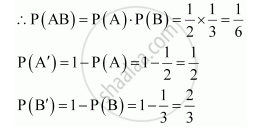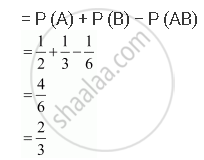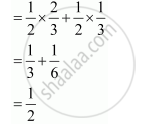# Probability of Solving Specific Problem Independently by a and B Are 1/2 and 1/3 Respectively. If Both Try to Solve the Problem Independently, Find the Probability that (I) the Problem is Solved (Ii) Exactly One of Them Solves the Problem. - Mathematics

Probability of solving specific problem independently by A and B are 1/2 and 1/3 respectively. If both try to solve the problem independently, find the probability that

(i) the problem is solved (ii) exactly one of them solves the problem.

#### Solution

Probability of solving the problem by A, P (A) =1/2

Probability of solving the problem by B, P (B) =1/3

Since the problem is solved independently by A and B,1) Probability that the problem is solved = P (A ∪ B)2)Probability that exactly one of them solves the problem is given by,    P(A).P(B') + P(B).P(A')Concept: Independent Events
Is there an error in this question or solution?

#### APPEARS IN

NCERT Class 12 Maths
Chapter 13 Probability
Q 14 | Page 547